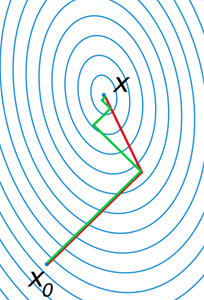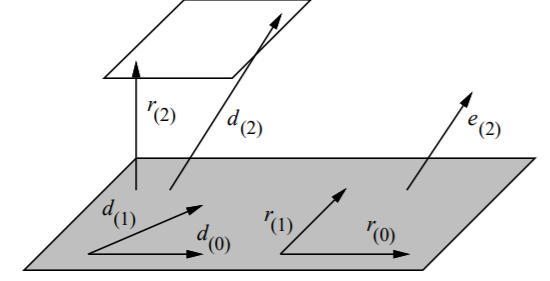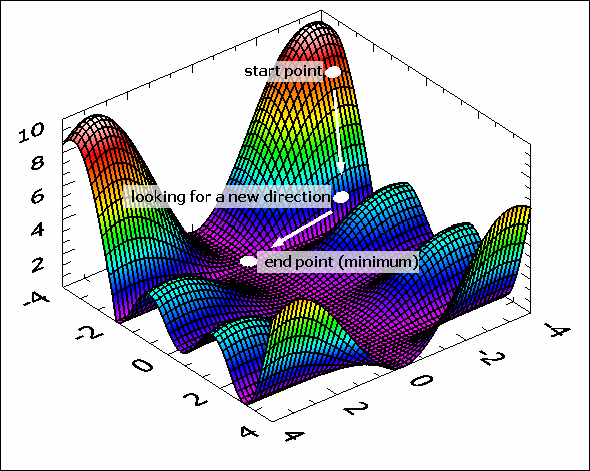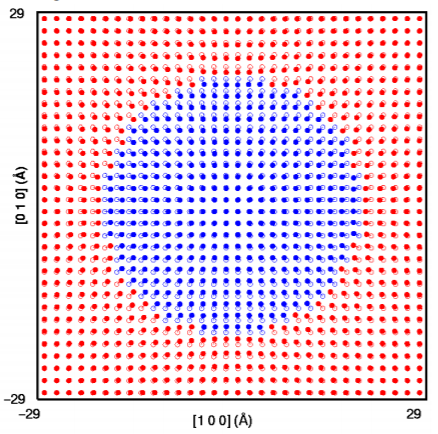# Conjugate Gradient Method and it’s Application in Energy Minimization

With the Covid-19 pandemic consuming the world, researchers are racing to find a drug that can help. One of the possibilities for such a drug is a small molecule. Finding such a drug by experiments alone is like finding a needle in a haystack. However, simulations can greatly improve scanning millions of “small-molecule” drug-like compounds to determine if any of them will work. A first step in drug discovery involves generating numerous conformations for each molecule and then finding the lowest energy. The resulting minimized conformation with respect to the potential energy then needs to be “docked” into the binding site of the protein to calculate the binding affinity.

The step of finding the most stable structure of each molecule, namely, the minimized conformation with the lowest energy can be achieved best through conjugate gradient method.

# What is the Conjugate Gradient Method

Conjugate gradient (CG) is an iterative solution for large systems of linear equations with the form Ax = b where A is a known square, symmetric, positive-indefinite matrix, b is a known vector, x is an unknown vector. Extending this concept to the energy minimization problem, let vector x = (r1x, r1y, r1z, r2x, r2y, r2z,…) be the set of atomic coordinates of n atoms that make up the molecule. For a given molecule, as the coordinates in x vary, the system potential energy V also changes. The energy minimization goal is then to find x such that V is a minimum. In the CG method the interatomic force, gradient of the function, is used to aid in finding the minimum. The gradient will always point in the direction of largest increase.

Consider an n x n matrix A and x and b are vectors,

A is positive-definite if, for every nonzero vector x,

and,

holds true. Since A is symmetric \$A^T = A\$,

Consider the plot of f(x) shown below:

The minimum point of this surface is the solution to Ax = b.

This can be solved by a direct method when n is small. However, for large n, the direct method will be too computationally heavy. Instead, conjugate gradient uses an iterative procedure to approximately solve systems where n is large.

“The name ‘Conjugate Gradients’ is a bit of a misnomer, because the gradients are not conjugate, and the conjugate directions are not all gradients. ‘Conjugated Gradients’ would be more accurate.” — Shewchuk

Let \$x_*\$ be the coordinates of the unknown minimum value of f(x) and \$x_0\$ be the initial guess value.

Starting with \$x_0\$ we search for the solution and in each iteration, we need a metric to tell us whether we are closer to the solution \$x_*\$. This metric comes from the fact that the solution \$x_*\$ is also the unique minimizer of the following quadratic function:

Let there be a set of mutually conjugate n vectors with respect to A denoted by P as follows:

Then the first basis vector \$p_0\$ is the negative of the gradient of f at x = \$x_0\$. The initial guess is \$p_0= bAx_0\$

The other vectors, including \$p_k\$ for the kth step in the basis will be conjugate to the gradient, hence the name conjugate gradient method.

The error:

is a vector that indicates how far we are from the solution. The residual indicates how far we are from the correct value of b. Initially this residual is \$p_0\$ and \$r_k\$ is the residual at the kth step:

The residual is the error transformed by A into the same space as b. \$r_k\$ is the negative gradient of f at x = \$x_k\$.

Therefore, the conjugate gradient method requires the next move to be in the direction \$r_k\$. In addition, the directions \$p_k\$ must be conjugate to each other. The way this can be enforced is by requiring that the next search direction should be built out of the current residual and all previous search directions. This results in the following:

The next optimal location is given by:

where,

Substituting for \$x_k+1\$ into f and minimizing it w.r.t. \$α_k\$ we arrive at

As shown above the iterative CG method uses knowledge of previous search directions and residual vectors.

## Visual Explanation of CGFigure 1. Convergence of gradient descent with optimal step size (green) and conjugate vector (red) for minimizing a quadratic function associated with a given linear system. Note: x is \$x_*\$

The conjugate gradient method was created by Hestenes and Stiefel around 1952. CG is a mathematical technique useful for optimizing both linear and non-linear systems. Generally, this technique is implemented iteratively, but can be utilized as a direct optimization method, and will return a numerical solution. CG is best utilized for finding the minimum of very large systems where solving the system with the direct method is not practical.

The subspace created by repeatedly applying a matrix to a vector is called the Krylov subspace. An example of Krylov subspace is shown below:Figure 2. In Conjugate Gradient method the new residual is orthogonal to all the previous residuals and search directions. The new search direction, d2, is a linear combination of r2 and d1.

The algorithm for CG is shown here. Given the inputs A, b, starting value x, a maximum number of iterations \$i_{max}\$, and an error tolerance ε < 1

The iteration stops either when the maximum number of iterations \$i_{max}\$ is reached or when the error tolerance is reached.

A simple python implementation of CG is given below:

# CG Applied to Energy Minimization

## Mathematics Behind Energy Minimization

CG method is used for energy minimization or geometry optimization in computational chemistry. The goal of this exercise is to get a set of coordinates for the atoms in a molecule which has lowest potential energy which is shown in Figure 3. The z-axis represents the potential energy and the goal is to arrive at the minimum energy configuration of the molecule.Figure 3. Shows a start point and a start direction. New points and new directions are calculated by the Conjugate Gradient

A simple but crude method for energy minimization is the Steepest Descent method where each step is in the direction of the gradient and eventually settle into a local minimum structure. Most modern energy minimization programs use more advanced procedures like Conjugate Gradient. An important aspect of advanced methods not implemented in Steepest Descent is using knowledge of the step history to accelerate the convergence to the minimum point.

While CG is a highly efficient method to finding a minimum, it does not have anything specific to atomistic simulation. A variation of CG called adaptive CG (ACG) is used for faster optimization.

The algorithm for the energy minimization using ACG is shown below:

## Real-World Examples of CG

Diabetes Drug: As mentioned at the beginning of the blog, energy minimization is extremely important in drug discovery, whether it is the protein structure or a small-molecule drug. CG for energy minimization has been used for decades in drug discovery and is being recently applied for Covid-19 drug candidates. Four drug molecules for Type 2 diabetes are listed in Table 1. The energy minimization in this case involves geometry optimization since there are several conformations possible due to the presence of rotatable bonds.

Consistently, Conjugate Gradient results in lower energy values for all molecules considered. Irrespective of the number of freely rotatable bonds in each of the molecule, Conjugate Gradient procedure is the method of choice for minimizing the energy of a molecule.

Cobalt-Copper Nanostructure: Cobalt in Copper has some desirable magnetic properties in the nanocrystalline regime. In order to perform simulations, the first step is to get a good molecular model of these nanostructures. In order to achieve this, one needs to perform energy minimization to get a reasonable structure before simulating the magnetic properties. Figure 4 shows such a model of Cobalt in Copper nanostructureFigure 4. Cobalt (blue) in Copper (red). Open circles are the original atom position and the closed circles are after energy minimization.

The energy minimization results are shown in the table for Steepest Descent and Conjugate Gradient. The number of iterations (No. of Iter.) and the energy tolerance (E. Tol.) are listed in Table 2.

Comparison of the two methods clearly shows that Conjugate Gradient is much better than Steepest Descent. For the same energy tolerance of 0.001, Conjugate Gradient needs only 27 iterations to reach the energy minimum in just 363.03 seconds while Steepest Descent not only takes much longer, it also does not arrive at the lowest energy.

# Conclusion

Conjugate Gradient method is an iterative gradient descent algorithm for finding the minimum value of a function. It’s most relevant application is energy minimization since it rapidly solves large systems of linear equations with an unknown minimum. CG’s iterative method takes vanilla gradient descent to the next level by using less computational resources and less iterations to optimize.

# References

http://www.acclab.helsinki.fi/~aakurone/atomistiset/lecturenotes/lecture12_2up.pdf